### Home > MC2 > Chapter 7 > Lesson 7.1.6 > Problem7-60

7-60.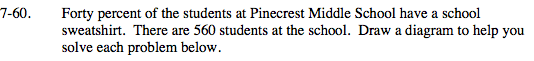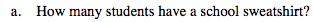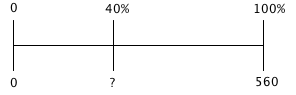$\frac{40}{100}\left(\frac{?}{?}\right)=\frac{\textit{x}}{560}$

x = 224 students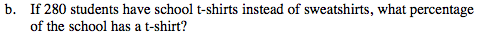280 is what percent of 560?

$\frac{\textit{x}}{100}\left(\frac{?}{?}\right)=\frac{280}{560}$

x = 50%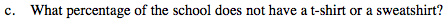If 40% of the students have a school sweatshirt and 50% have a t-shirt instead of a sweatshirt, what is the remaining percentage of students that have neither?